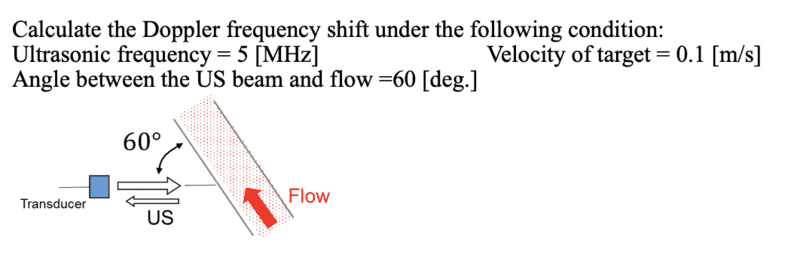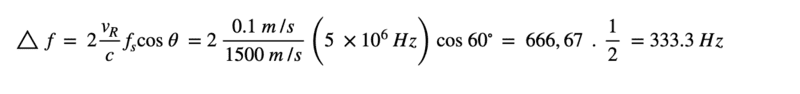# Doppler frequency shift

• nao113

#### nao113

Homework Statement
Calculate the Doppler frequency shift under the following condition:
Ultrasonic frequency = 5 [MHz] Velocity of target = 0.1 [m/s]
Angle between the US beam and flow =60 [deg.]

I put the detait of the question and my answer below
Relevant Equations
f = 2. vr. fs. cos /c•Delta2

Homework Statement:: Calculate the Doppler frequency shift under the following condition:
Ultrasonic frequency = 5 [MHz] Velocity of target = 0.1 [m/s]
Angle between the US beam and flow =60 [deg.]

I put the detait of the question and my answer below